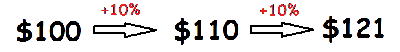Math Calculators, Lessons and Formulas

It is time to solve your math problem

mathportal.org

# Compound interest calculator

Compound Interest is calculated on the initial payment and also on the interest of previous periods.

Example: Suppose you give \$100 to a bank which pays you 10% compound interest at the end of every year. After one year you will have \$100 + 10% = \$110, and after two years you will have \$110 + 10% = \$121.Problem: How much money would you need to deposit today at 8% annual interest compounded monthly to have$1200 in the account after 12 years?

Result:

The principal is 460.72. Explanation: To find amount we use formula:  $$A = P \left( 1 + \frac{r}{n} \right)^{\Large{n \cdot t}}$$ A = total amount P = principal or amount of money deposited, r = annual interest rate n = number of times compounded per year t = time in years In this example we have $$A = \1200 ~,~ r = 8 \% ~ , ~ n = 12 ~ \text{and} ~ t = 12 ~ \text{years}$$ After plugging the given information we have \begin{aligned} 1200 &= P \left( 1 + \frac{ 0.08 }{ 12 } \right)^{\Large{ 12 \cdot 12 }} \\ 1200 &= P \cdot { 1.00667 } ^ { 144 } \\ 1200 &= P \cdot 2.604631 \\ P &= \frac{ 1200 }{ 2.604631} \\ P &= 460.72 \end{aligned} ## Report an Error ! Script name : compound-interest-calculator Imputed values: 1 , 1 , 8 , 12 , 1 , 4 , 1200 Comment (optional) Email (optional) ## Share Result Share this result with others by using the link below. You can click here to verify link or you can just copy and paste link wherever you need it. Compound Interest Calculator How much one will get after investment time. show help ↓↓ examples ↓↓ 0123456789.del Hide steps Compound Interest Calculator Here you can select a value you want to find. show help ↓↓ examples ↓↓ 0123456789.del Hide steps working... examples example 1:ex 1: What will a deposit of\color{blue}{\$4500}$ at $\color{blue}{7\%}$ compounded $\color{blue}{\text{yearly}}$ interest be worth if left in the bank for $\color{blue}{\text{9 years}}$ ?
example 2:ex 2:
What will a deposit of $\color{blue}{\$3500}$at$\color{blue}{10\,\%}$compounded$\color{blue}{\text{monthly}}$be worth if left in the bank for$\color{blue}{8 \, \text{years}}$? example 3:ex 3: How much money would you need to deposit today at$\color{blue}{8\% \, \text{annual}}$interest compounded$\color{blue}{\text{monthly}}$to have$\color{blue}{\$1200}$ in the account after $\color{blue}{12 \, \text{years}} \, \text{?}$
example 4:ex 4:
Find the present value of $\color{blue}{\$1000}$to be received at the end of$\color{blue}{2 \, \text{years}}$at a$\color{blue}{12\%}$nominal annual interest rate compounded$\color{blue}{\text{quarterly}}$. example 5:ex 5: What annual interest rate is implied if you lend someone$\color{blue}{\$1700}$ and are repaid $\color{blue}{\$ 1910}$in$\color{blue}{\text{two years}}$? example 6:ex 6: Suppose that a savings account is compounded$\color{blue}{\text{monthly}}$with a principal of$\color{blue}{\$1350}$. After $\color{blue}{8 \, \text{months}}$, the amount increased to $\color{blue}{\$ 1424}$. What was the per annum interest rate? example 7:ex 7: How long does it take for$\color{blue}{\$4300}$ to grow into $\color{blue}{\$6720}$at$\color{blue}{9\,\%}$compounded$\color{blue}{\text{quarterly}}\$?

Quick Calculator Search